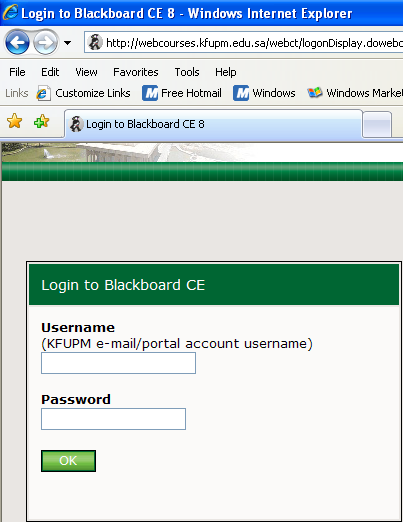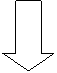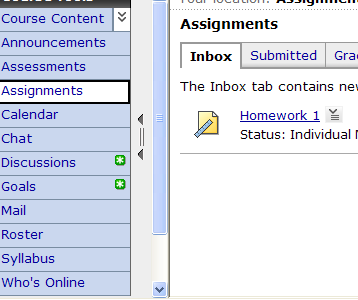ICS 101 - Computer programming

Lab # 6

( Functions ) II

Objectives

•    Special Cases of Functions

Intrinsic (built-in) Functions

 Function Function Value Comment SQRT(X) Square Root of X X is a real argument ABS(X) Absolute Value of X INT(X) Integer Value of X Converts a real to an integer REAL(K) Real Value of X Converts  an integer to a real MOD(M, N) M - M / N * N Modulo function

Statement Functions

Syntax

where

fname is the name of  the function

a list of arguments is the optional list of dummy arguments

expression computes the function valueExercise 1

Write a program which prompts for and reads a positive integer N. It then calls a logical statement function MLTPL3 which returns the value .TRUE . if the integer N is divisible by 3. The program then prints one of the following messages DIVISIBLE BY 3 or NOT DIVISIBLE BY 3 accordingly.

Exercise 2

Write a function that takes as input three integer numbers, it then returns the minimum number. Write a main program to test your function.

Exercise 3    (Homework) DO this exercise and submit in webCT

Write a function subprogram COST that computes the cost of postage according to the following: 2.50 Riyals for any weight equal to or less than a kilogram. Every additional kilogram is charged 3.0 Riyals , plus 100 Riyals extra charge If the customer wants fast delivery. The arguments to the function are the weight of the package and a logical variable FAST indicating fast delivery. Write a main program to test the function.